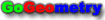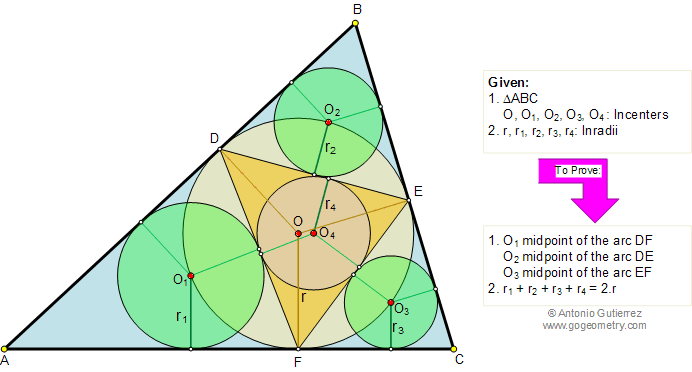Problem 209. Triangle, Incircles, Inradius, Auxiliary lines
 The figure shows a triangle ABC with the contact triangle DEF. O, O1, O2, O3, and O4 are the incenters of triangles ABC, ADF, BDE, CEF, and DEF, and r, r1, r2, r3, and r4 are the inradii, respectively. Prove (1) O1, O2, O3 are the midpoints of the arcs DF, DE, and EF respectively, and (2) r1 + r2 + r3 + r4 = 2.r View or post a solution.Home | Geometry | Search | Problems | 201-210 | Email | View or post a solution